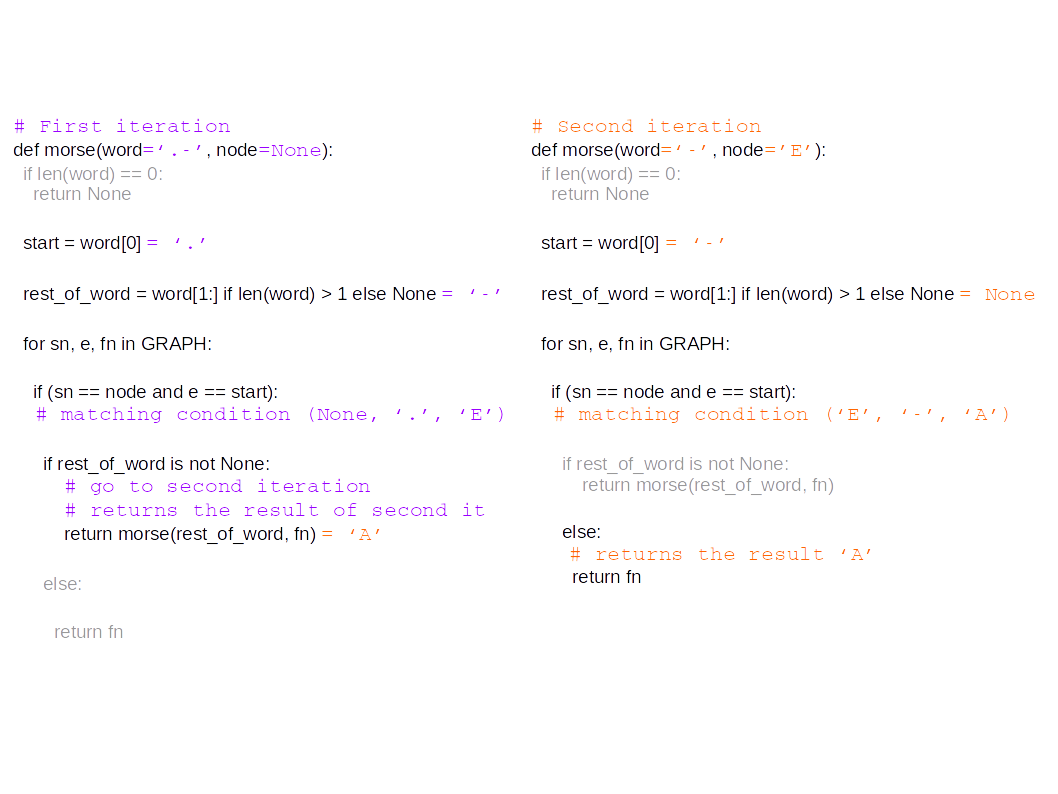# Challenge

The challenge was the following:

Given a “morse” code following the rule describe on the image below:For instance:

• ”.” = “E”
• ”..” = “I”
• ”-.-“ = “K”

You will be given a “word” made of up to three symbols. Some symbols may be missing and replaced with a question mark “?”. You have to determine all possible letters matching the word. For instance, if given “?”, the program should return “[‘E’, ‘T’]”. For the input word “.?”, it should return “[‘I’, ‘A’]”.

Wow wow wow. That was my first reaction. Then I thought of the work I have been doing on graphs for the past few weeks and the following idea came to my mind.

# My solution

This exercice makes me think of a graph structure, with path search. So, I started by creating a graph, where each edge is defined by a starting node (letter at level l), ending node (letter at level l+1) and the symbol between them:

## Exploring the graph with no missing letter

With this graph structure, you can see that, for instance, “..” corresponds to “None POINT ‘E’ POINT ‘I’” = ‘I’. Let’s write the corresponding code to “read” the graph:

Code that can be tested with:

if __name__ == "__main__":
print(morse("..")) # 'I'


Here is a picture illustrating what is going on:## Extending previous code to take into account missing letters

Our code should now return a list of possiblities instead of a single letter. Here are the changes to the previous version in order to make it work:

And, as usual, an example usage of this function:

if __name__ == "__main__":
print(morse2("?.")) # ['I', 'N']


Works like a charm! I love graphs!